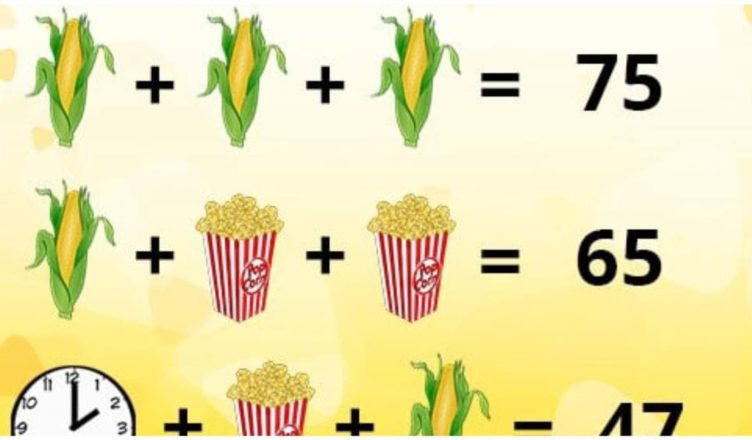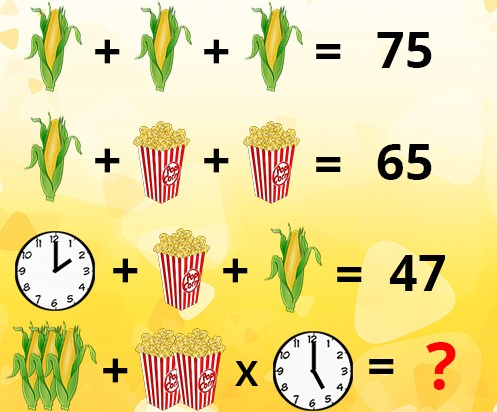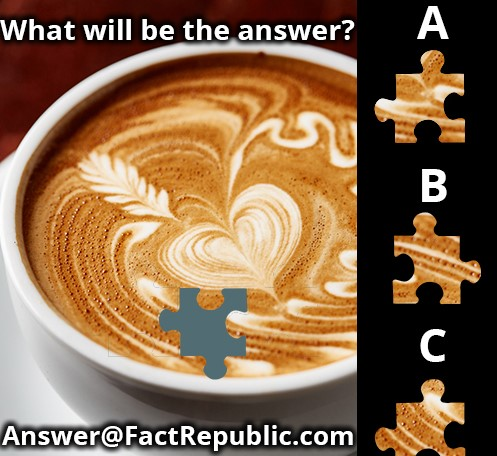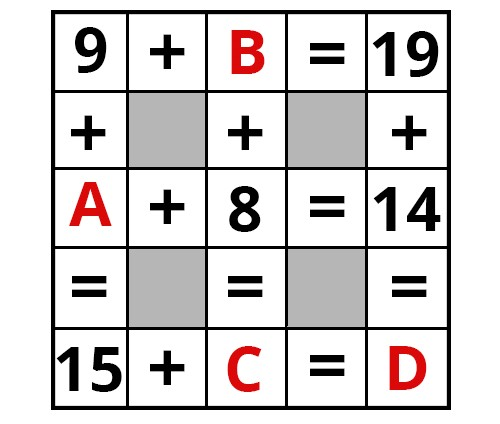# Brain Test. Popcorn PuzzleWe are providing some basic points for solving puzzles. Requesting you to go through the below points before taking any online test. Reasoning puzzles are favorite questions in all public examinations. This puzzles questions challenge you to match the correct answers.

Some time it’s takes long time to solve questions. Even though we will solve it. But it is recommended to skip the question if you are not able to solve the question with in less than two or max three minutes otherwise you are in trouble which leads to time insufficient!!!Corn = 25. (25+25+25 = 75). Popcorn = 20. (25+20+20 = 65). Clock face with 2 o’clock = 2. (2+20+25 = 47). So final equation will be 75 + 40 x 5 = 75 + 200 = 275. 75 because there are 3 corns. 40 because there are two popcorn tubs. 5 because the clock face reads 5 o’clock.